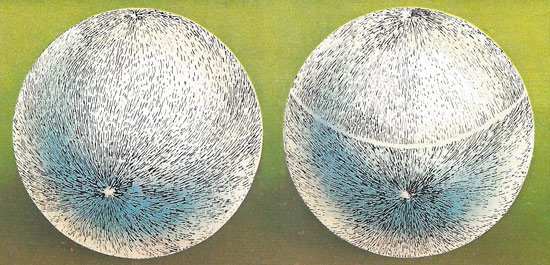A# hairy ball theoremA ball covered with fur cannot be smoothed down all over; at least two crowns must be remain where the fur radiates from a point or piles up at one, or a set of partings. This "hairy ball" theory governs the ways directions, like hairs, can be aligned on a sphere. If they are lines of magnetic flux, it shows that every magnet must have two poles. If they are wind directions on the globe, the theory proves that somewhere in the world the wind is not blowing.

The hairy ball theorem asserts that if a sphere is covered with hair or fur, like a tennis ball, the hair cannot be brushed so that it lies flat at every point. In mathematical terms: any continuous tangent vector field on the sphere must have a point where the vector is zero. This theorem also means that somewhere on Earth's surface there has to be a point where the horizontal wind speed is zero, even if it's windy everywhere else.

Does the same apply to a torus? Is there a hairy donut theorem? No! The number of 'problem points', where the hair would stick up on a surface, is related to a quantity called the Euler characteristic of that surface. Basically, every point on a surface has an index that describes how many times the vector field rotates in a neighborhood of the problem point. The sum of the indices of all the vector fields is the Euler characteristic. Since the torus has Euler number 0, it is possible to have a covering of hair – a vector field – on it that lies flat at every point.